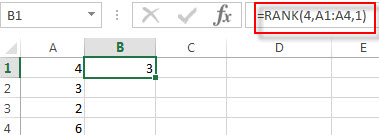# Excel RANK Function

This post will guide you how to use the RANK function with syntax and examples in Microsoft excel.

### Description

The Excel RANK function returns the rank of a given number in a supplied range of cells.  If there are duplicated values in a list of values, it will be set the same rank. This function has been replaced by the RANK.EQ function in Excel 2010.

The RANK function is a build-in function in Microsoft Excel and it is categorized as a Statistical Function.

The RANK function is available in Excel 2016, Excel 2013, Excel 2010, Excel 2007, Excel 2003, Excel XP, Excel 2000, Excel 2011 for Mac.

### Syntax

The syntax of the RANK function is as below:

`= RANK (number,ref,[order])`

Where the RANK function arguments are:

• Number – This is a required argument.  The number for which you want to get the rank.
• ref – This is a required argument. An array or range of cells, or cell reference that contain the supplied number.
• Order – This is an optional argument. . A number specifying how to rank number. If order is 0 or omitted, the RANK function will rank the numbers in descending order. If the order is not equal to 0, the RANK function will rank numbers in ascending order.

### Excel RANK Function Examples

The below examples will show you how to use Excel RANK Function to get the rank of a given value within a supplied range of cells.

Example 1:  to get the rank of 4 in a given range of cells (A1:A4), using the following formula:

`=RANK(5,A1:A4,1)`### More Excel Rank Function Examples

• Rank Data with Multiple Criteria
Assuming that you want to rank data against multiple criteria for a range of cells in your worksheet. How to achieve it. You need to create a new complex formula based on the SUMPRODUCT function. .…
•  Rank values in a column based a specific value in another column
Assuming that you have a list of data contains two columns and the first column is product list and another is Sales number. You want to rank the sales number of a specified product name. You can try to write a complex formula based on the IF function and the COUNTIFS function to achieve the result..…
• Reverse Rank Order
Assuming that you have a formula based on RANK function that ranks numbers by biggest to smallest by default. But you want to go the opposite from the smallest to biggest.…
• Rank data Based on the Number of Occurrences
You need to get the number of occurrences for the selected range of cells, then rank those number using the RANK function…